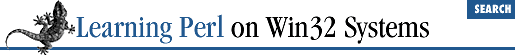home | O'Reilly's CD bookshelfs | FreeBSD | Linux | Cisco | Cisco Exam

### 6.3 Output to STDOUT

Perl uses the ``` print``` and ``` printf``` functions to write to standard output. Let's look at how they are used.

### 6.3.1 Using print for Normal Output

We've already used ``` print``` to display text on standard output. Let's expand on that usage a bit.

The ``` print``` function takes a list of strings and sends each string to standard output in turn, without any intervening or trailing characters added. What might not be obvious is that ``` print``` is really just a function that takes a list of arguments, and returns a value like any other function. In other words:

`\$a = print("hello ", "world", "\n");`

would be another way to say ``` hello world``` . The return value of ``` print``` is a true or false value, indicating the success of the print. The print nearly always succeeds, unless you get some I/O error, so ``` \$a``` in this case will usually be 1.

Sometimes you'll need to add parentheses to ``` print``` as shown in the example given below, especially when the first thing you want to print starts with a left parenthesis, as in:

```print (2+3),"hello";   # wrong! prints 5, ignores "hello"
print ((2+3),"hello"); # right, prints 5hello
print 2+3,"hello";     # also right, prints 5hello```

### 6.3.2 Using printf for Formatted Output

You may wish to have a little more control over your output than ``` print``` provides. In fact, you may be accustomed to the formatted output of C's ``` printf``` function. Fear not: Perl provides a comparable operation with the same name.

The ``` printf``` function takes a list of arguments (enclosed in optional parentheses, like the ``` print``` function). The first argument is a format control string, defining how to print the remaining arguments. Here's an example:

` printf "%15s %5d %10.2f\n", \$s, \$n, \$r;`

This function prints ``` \$s``` in a 15-character field, then a space, then ``` \$n``` as a decimal integer in a 5-character field, then another space, then ``` \$r``` as a floating-point value with 2 decimal places in a 10-character field, and finally a newline.

Among the many formats supported by Perl's ``` printf()``` and ``` sprintf()``` functions are the following commonly used ones:

 %% Percent sign %c Character with the given number %s String %d Signed integer, in decimal %u Unsigned integer, in decimal %o Unsigned integer, in octal %x Unsigned integer, in hexadecimal %e Floating-point number, in scientific notation %f Floating-point number, in fixed decimal notation %g Floating-point number, in %e or %f notation

Between the percent and the format character, you may place one or more of the following flags:

 space Prefix positive number with a space + Prefix positive number with a plus sign - Left justify within the field 0 Use zeros, not spaces, to right justify number Minimum field width .number Precision: digits after decimal point for floating-point number, maximum length for string, minimum length for integer6.2 Input from the Diamond Operator6.4 Exercises